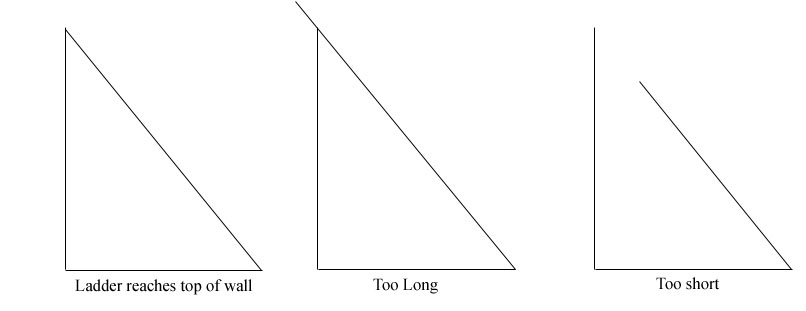SEARCH HOMEMath Central Quandaries & Queriesclinton, a parent: a ladder rests against a wall 24m high.the foot of the ladder is 7m from the foot of the wall. How do you calculate the length of the ladderProblems like this often have some unstated assumptions.
Here, you have to assume that the ladder reaches exactly to the top of the wall, otherwise the answer could be anything from 7m up!You also assume the wall is vertical and the ground horizontal so that there is a right angle between them.

So you have a right triangle with base 7 and altitude 24.
Use Pythagoras' theorem

a2 + b2 = h2

to find the length of the ladder.

Good Hunting!

- RDMath Central is supported by the University of Regina and The Pacific Institute for the Mathematical Sciences.